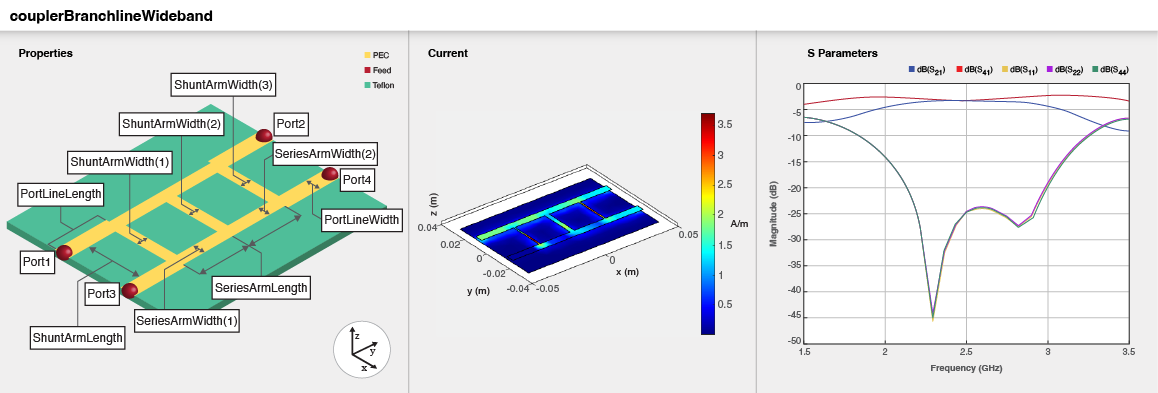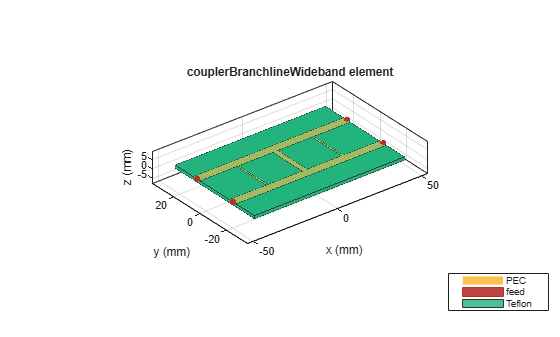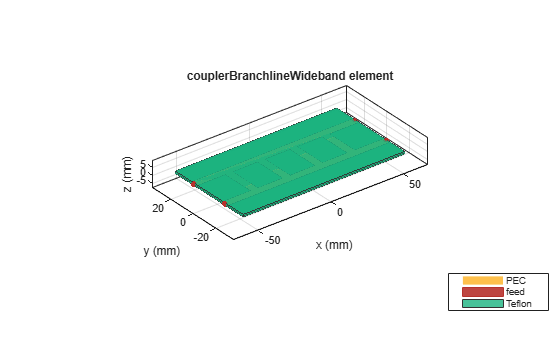# couplerBranchlineWideband

Create equal split multisection branchline coupler or quadrature hybrid

Since R2023a

## Description

Use the `couplerBranchlineWideband` object to create an equal split multi-section branchline coupler or quadrature hybrid.To analyze the behavioral model for the branchline coupler, set the `Behavioral` property in the `sparameters` function to `true` or `1`.

## Creation

### Syntax

``coupler = couplerBranchlineWideband``
``coupler = couplerBranchlineWideband(Name=Value)``

### Description

example

````coupler = couplerBranchlineWideband` creates a equal split multisection branchline coupler with properties for a frequency of 2.5 GHz.```

example

````coupler = couplerBranchlineWideband(Name=Value)` sets Properties using one or more name-value arguments. For example, `couplerBranchlineWideband(NumSections=4)` creates a branch line coupler with four sections. Properties not specified retain their default values.```

## Properties

expand all

Number of sections, specified as a positive scalar. The minimum number of sections you can specify is two and the maximum is six.

Example: `coupler = couplerBranchlineWideband(NumSections=4)`

Data Types: `double`

Length of the input and the output line in meters, specified as a positive scalar.

Example: ```coupler = couplerBranchlineWideband(PortLineLength=0.0286)```

Data Types: `double`

Width of the input and the output line in meters, specified as a positive scalar.

Example: ```coupler = couplerBranchlineWideband(PortLineWidth=0.0070)```

Data Types: `double`

Length of the series arm in meters, specified as a positive scalar.

Example: ```coupler = couplerBranchlineWideband(SeriesArmLength=0.0286)```

Data Types: `double`

Width of the series arm in meters, specified as a positive scalar or a vector.

Example: ```coupler = couplerBranchlineWideband(SeriesArmWidth=0.0096)```

Data Types: `double`

Length of the shunt arm in meters, specified as a positive scalar.

Example: ```coupler = couplerBranchlineWideband(ShuntArmLength=0.0286)```

Data Types: `double`

Width of the shunt arm in meters, specified as a positive scalar or a vector.

Example: ```coupler = couplerBranchlineWideband(ShuntArmWidth=0.0096)```

Data Types: `double`

Height of the branchline coupler from the ground plane in meters, specified as a positive scalar.

In a multilayer substrate, you can use the `Height` property to create a branchline coupler where the two dielectrics interface.

Example: `coupler = couplerBranchlineWideband(Height=0.0076)`

Data Types: `double`

Width of the ground plane in meters, specified as a positive scalar.

Example: ```coupler = couplerBranchlineWideband(GroundPlaneWidth=0.046)```

Example: double

Type of dielectric material used as a substrate, specified as a `dielectric` object. The default height of the substrate is `0.0016` meters. The dielectric material in a `couplerBranchlineWideband` object with default properties is Teflon.

Example: `d = dielectric("FR4");` `coupler = couplerBranchlineWideband(Substrate=d)`

Type of metal used in the conducting layers, specified as a `metal` object. The metal used in the conducting layers of a `couplerBranchlineWideband` object with default properties is PEC.

Example: `m = metal("Copper");` `coupler = couplerBranchlineWideband(Conductor=m)`

Data Types: `string` | `char`

## Object Functions

 `charge` Calculate and plot charge distribution `coupling` Calculate coupling factor of coupler `current` Calculate and plot current distribution `dgs` Create defected ground structure of PCB element `design` Design wideband branchline coupler around particular frequency `directivity` Calculate directivity of coupler `feedCurrent` Calculate current at feed port `isolation` Calculate isolation of coupler `layout` Plot all metal layers and board shape `mesh` Change and view mesh properties of metal or dielectric in PCB component `shapes` Extract all metal layer shapes of PCB component `show` Display PCB component structure or PCB shape `sparameters` Calculate S-parameters for RF PCB objects

## Examples

collapse all

Create a default wideband branchline coupler.

`coupler = couplerBranchlineWideband`
```coupler = couplerBranchlineWideband with properties: NumSections: 2 PortLineLength: 0.0223 PortLineWidth: 0.0051 SeriesArmLength: 0.0223 SeriesArmWidth: 0.0051 ShuntArmLength: 0.0223 ShuntArmWidth: [9.6000e-04 0.0029 9.6000e-04] Height: 0.0016 GroundPlaneWidth: 0.0600 Substrate: [1x1 dielectric] Conductor: [1x1 metal] ```

View the coupler.

`show(coupler)`Create a default two-section wideband branchline coupler and visualize it.

```coupler = couplerBranchlineWideband; show(coupler);```Calculate the coupling at 2.5 GHz.

`c = coupling(coupler,2.5e9)`
```c = -3.1935 ```

```coupler = couplerBranchlineWideband(NumSections=3,ShuntArmWidth=0.0051); coupler.Substrate = dielectric(Name={'Teflon','Teflon'},EpsilonR=[2.1 2.1], ... LossTangent=[0 0],Thickness=[0.8e-3 0.8e-3]); coupler.Height = 0.8e-3;```
`show(coupler)`